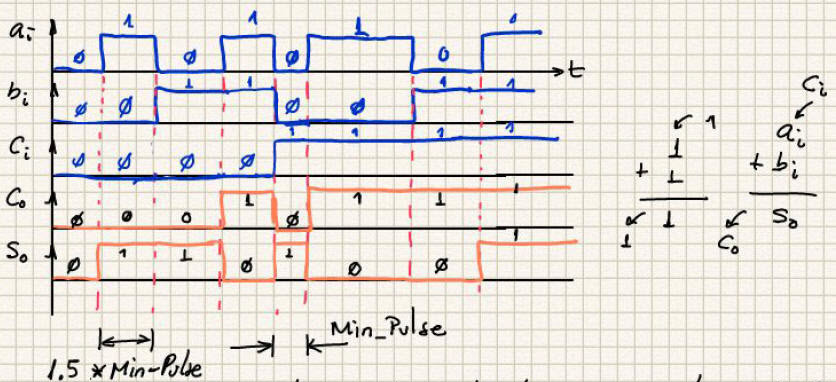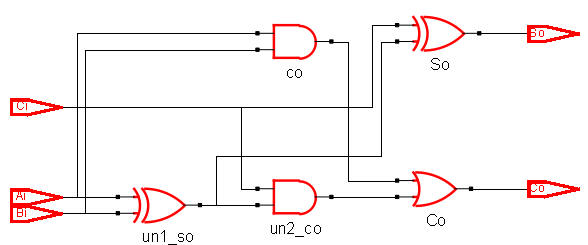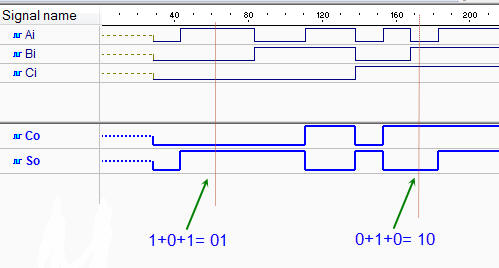﻿ Digital Circuits and Systems - Circuits i Sistemes Digitals (CSD) - EETAC - UPCBachelor's Degree in Telecommunications Systems and in Network Engineering## Adder_1bit plan A: structural single-file VHDL

Structural design of a 1-bit full adder circuit  (Plan A)

1. Specifications

Design a 1-bit adder using structural equations.Fig. 1. Symbol and truth table of a 1-bit adder circuit. It is also called full adder.

This in Fig. 2 is a simple sketch that represents the truth table in time. The idea of applying input vectors that can be any binary combination.Fig. 2. Timing diagram where all the inputs are applied sequentially and hence, the output is predicted accordingly to the truth table.

2. Planning

The VHDL file will be named Adder_1bit.vhd, the same name given to the entity. The project name is Adder_1bit_prj.

The block's architecture can be derived from equations (plan A). Thus, consider a broad view of the problem. This is a list of planning examples, and the list index is giving you an idea on how to name the corrsponding project folder:

Plan A ) Structural using equations (flat, single file project):

A1_M.- Truth table represented as a product of maxterms (canonical circuit)

A2_m.- Truth table represented as a sum of minterms (canonical circuit).

A3_SoP.- Simplified equations using Minilog.exe: SoP.

A4_PoS.- Simplified equations using Minilog.exe: PoS.

A5_NOR.- It is also possible to modify any of previous equations in order to use only-NOR gates.

A6_NAND.- It is also possible to modify any of previous equations in order to use only-NAND gates.

A7.- Any other equation. For instance, this is an implementation realising that XOR gates can be used to replace some AND and OR gates, which makes a simpler circuit. And . is a video recording of the project.

3. Developing the project using EDA tools

This is the translation to VHDL Adder_1bit.vhd of the 7th structural description from above. The project location is

Run a EDA project to synthesise the circuit. Fig. 3 shows an example of RTL schematic.Fig. 3. Example RTL when the project is developed  using some sort of equations like the ones written in plan A7.

4. Testing and validating the design

An example test bench Adder_1bit_tb.vhd. Run the VHDL simulation EDA tool to obtain and discuss the timing diagram.  Remember that from the testing point of view, even if you have designed the Adder_1bit using several plans, you can use all the time the same testbench.Fig. 4. Example testbench showing results

5. Report

Project report starting with the template sheets of paper, scanned figures, file listings, docx , pptx, or any other resources.

6. Prototyping

Use training boards and perform laboratory measurements to verify how the circuit works.

Other similar tutorial exercises

- Problem 3.11 in the CSD collection is for designing two chips: 1-bit subtractor (Subt_1bit) using equations, and 8-bit substractor (Subt_8bit) using a ripple-borrow hierarchical design. Some notes on its solution.